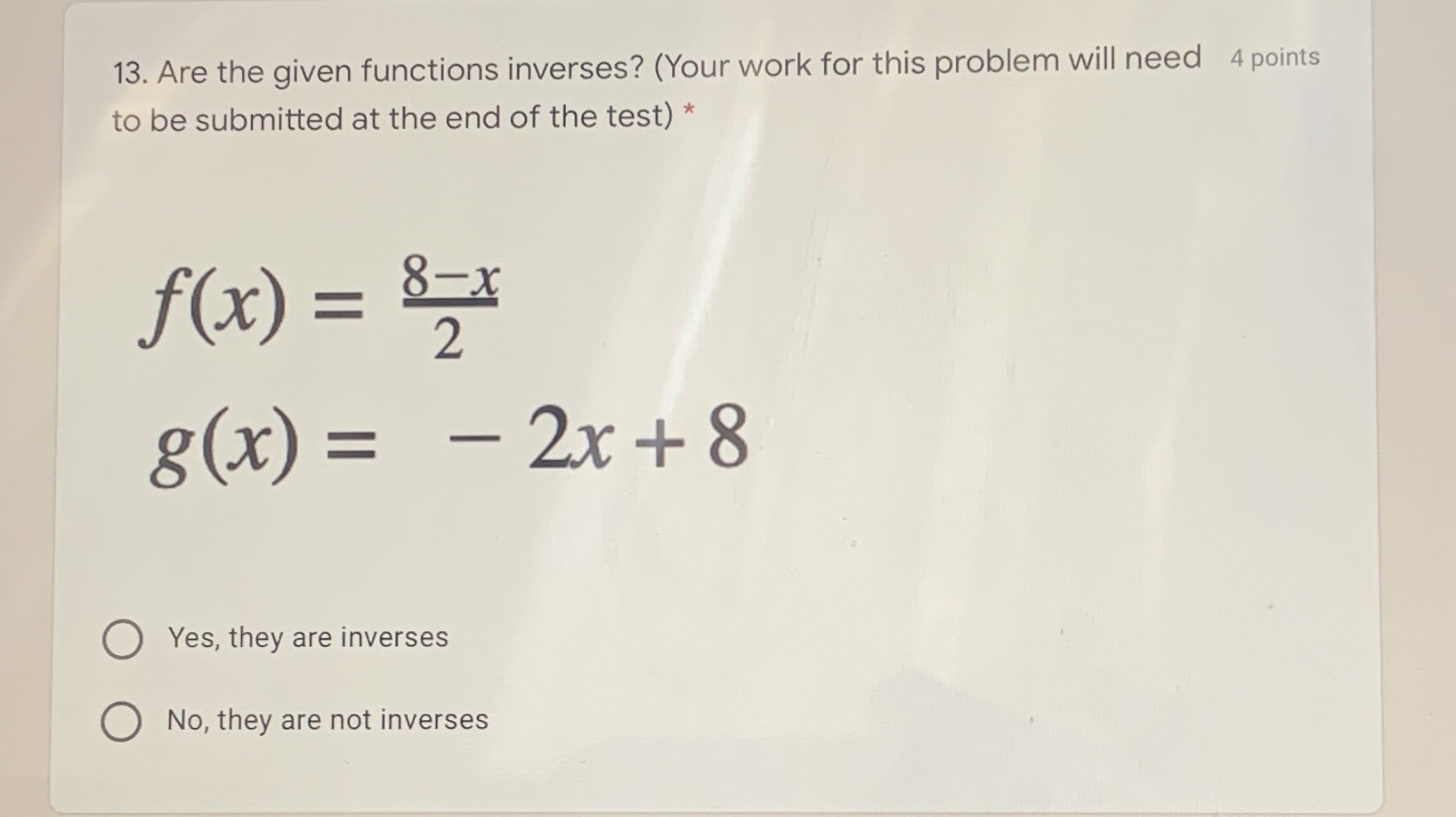### ¿Todavía tienes preguntas de matemáticas?

Pregunte a nuestros tutores expertos
Algebra
Pregunta13. Are the given functions inverses? (Your work for this problem will need $$4$$ points to be submitted at the end of the test) * $$f ( x ) = \frac { 8 - x } { 2 }$$ , $$g ( x ) = - 2 x + 8$$

Yes, they are inverses

No, they are not inverses